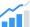# A Method to Measure Die Pad Capacitance

How to measure the chip die pad capacitance using time delay reflectometry (TDR).This paper defines a method to measure the chip die pad capacitance using time delay reflectometry (TDR). This method is useful for measuring the low-value capacitance that is present at the end of a transmission line.

In all protocol specifications, pad capacitance is an important electrical parameter to be measured because it directly affects the bandwidth. However, it is a challenge to measure the capacitance as it is a low value and not easily accessible for measurement. In addition, direct measurement may add some capacitance due to the PCB trace. Hence, a method is required to measure the capacitance accurately. This paper discusses a method to measure capacitance using TDR, which depends solely on the rise time, and uses the concept of transmission line.

This document introduces all the parameters and concepts that are required to understand this method of measuring die pad capacitance.